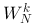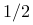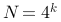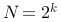14/05/16 10:26

The Goal

To try to see if performing a radix-16 step will improve execution time performance of a radix-4 based decimation in frequency (DIF) Fast Fourier Transform routine.

I will compare it to my other routines but I will also create another new but algorithmically comparable routine which just uses radix-4 steps so i'm not caught up in excessive optimisation.

As a secondary goal I intend to integrate all my knowledge gained over the last week and hopefully come up with a performant and scalable solution; but i don't know yet if i will fail at that.The Process

I will write this in the form of a 'live blog' of the development process as it happens.

I wont include any broken snippets of code but I will document them. I wont include boilerplate or other trivial routines apart from the complete source code at the end.

So in this way it is a sort of 'literate-light' document.

First the license, then time to begin.

This program is free software: you can redistribute it and/or modify
it under the terms of the GNU Affero General Public License as

This program is distributed in the hope that it will be useful,
but WITHOUT ANY WARRANTY; without even the implied warranty of
MERCHANTABILITY or FITNESS FOR A PARTICULAR PURPOSE.  See the
GNU Affero General Public License for more details.

You should have received a copy of the GNU Affero General Public License
along with this program.  If not, see http://www.gnu.org/licenses/.

14/05/16 10:48

Coffee in hand, lets go.

I will first write an all radix-4-pass implementation.

I have a couple of small modules I will copy in. One is a general purpose radix-4 step, and the other is a an fft of size 4 because it is so simple and worth including for the performance.

protected void radix4(float[] src, int i0, int nh, float[] w, int k) {
float a0r = src[i0 + nh * 0], a0i = src[i0 + nh * 0 + 1];
float a1r = src[i0 + nh * 1], a1i = src[i0 + nh * 1 + 1];
float a2r = src[i0 + nh * 2], a2i = src[i0 + nh * 2 + 1];
float a3r = src[i0 + nh * 3], a3i = src[i0 + nh * 3 + 1];

float a1rp3r = a1r + a3r, a1rp3i = a1i + a3i;
float a1rm3r = a1r - a3r, a1im3i = a1i - a3i;
float a0rp2r = a0r + a2r, a0ip2i = a0i + a2i;
float a0rm2r = a0r - a2r, a0im2i = a0i - a2i;

float b0r = a0rp2r + a1rp3r, b0i = a0ip2i + a1rp3i;
float b1r = a0rm2r + a1im3i, b1i = a0im2i - a1rm3r;
float b2r = a0rp2r - a1rp3r, b2i = a0ip2i - a1rp3i;
float b3r = a0rm2r - a1im3i, b3i = a0im2i + a1rm3r;

float w1r = w[k + 0], w1i = w[k + 1];
float w2r = w[k + 2], w2i = w[k + 3];
float w3r = w[k + 4], w3i = w[k + 5];

src[i0 + nh * 0 + 0] = b0r;
src[i0 + nh * 0 + 1] = b0i;
src[i0 + nh * 2 + 0] = b1r * w1r - b1i * w1i;
src[i0 + nh * 2 + 1] = b1i * w1r + b1r * w1i;

src[i0 + nh * 1 + 0] = b2r * w2r - b2i * w2i;
src[i0 + nh * 1 + 1] = b2i * w2r + b2r * w2i;
src[i0 + nh * 3 + 0] = b3r * w3r - b3i * w3i;
src[i0 + nh * 3 + 1] = b3i * w3r + b3r * w3i;

if (debug)
System.out.printf("%04x %04x W%04d Wk = %8.5f%+8.5fj  %8.5f%+8.5fj  %8.5f%+8.5fj\n", i0, nh, k,
w1r, w1i, w2r, w2i, w3r, w3i);
}
protected void radix4_0(float[] src, int i0, int nh) {
float a0r = src[i0 + 0];
float a2r = src[i0 + nh * 2];
float a1r = src[i0 + nh];
float a3r = src[i0 + nh * 3];

float a0i = src[i0 + 1];
float a2i = src[i0 + nh * 2 + 1];
float a1i = src[i0 + nh + 1];
float a3i = src[i0 + nh * 3 + 1];

float a1rp3r = a1r + a3r, a1rp3i = a1i + a3i;
float a1rm3r = a1r - a3r, a1im3i = a1i - a3i;
float a0rp2r = a0r + a2r, a0ip2i = a0i + a2i;
float a0rm2r = a0r - a2r, a0im2i = a0i - a2i;

src[i0 + 0] = a0rp2r + a1rp3r;
src[i0 + nh] = a0rp2r - a1rp3r;
src[i0 + nh * 2] = a0rm2r + a1im3i;
src[i0 + nh * 3] = a0rm2r - a1im3i;

src[i0 + 1] = a0ip2i + a1rp3i;
src[i0 + nh + 1] = a0ip2i - a1rp3i;
src[i0 + nh * 2 + 1] = a0im2i - a1rm3r;
src[i0 + nh * 3 + 1] = a0im2i + a1rm3r;

if (debug)
System.out.printf("%04x %04x     Wk = %8.5f%+8.5fj  %8.5f%+8.5fj  %8.5f%+8.5fj\n", i0, nh,
1., 0., 1., 0., 1., 0.);
}

These routines take indexes which are premultiplied, incase they can't be inlined.

The radix4 routine defines the 'twiddle factor' table format, so next is a routine which builds such a table.

synchronized static float[] getw(int logN) {
int N = 1 << logN;
float[] w;

if (wtables[logN] == null || (w = wtables[logN].get()) == null) {
w = new float[6 * N / 4];

for (int i = 0; i < N / 4; i++) {
w[i * 6 + 0] = (float) cos(-2.0 * PI * i / N);
w[i * 6 + 1] = (float) sin(-2.0 * PI * i / N);
w[i * 6 + 2] = (float) cos(-4.0 * PI * i / N);
w[i * 6 + 3] = (float) sin(-4.0 * PI * i / N);
w[i * 6 + 4] = (float) cos(-6.0 * PI * i / N);
w[i * 6 + 5] = (float) sin(-6.0 * PI * i / N);
}

wtables[logN] = new WeakReference<>(w);
}
return w;
}

Because i'm only implementing radix-4 stages this requires N/4 entries but covers all 3 exponents ofrequired. Because I want this code to be reusable and efficient i'm using a WeakReference to share the tables between instances and synchronising the call.

14/05/16 11:15

House things, washing, had a shower, made a bowl of porridge and got the coffee maker on the stove.

14/05/16 11:36

So where was i ...

Perhaps surprisingly, that is all the maths out of the way!

First I will create the `simpler' driver - one that just does radix-4 passes. I will however encode some foreknowledge about how to structure the code to enable multi-threading and some other performance improvements into the algorithm.

The algorithm will proceed in two stages:

1. Full passes across the whole data of size N ... M;
2. N/M passes of transforms of size M ... 4;

As it is a DIF algorithm it starts at size N and ends at size 4. Step 2 has two purposes, one is to improve locality of reference so it should be tuned relative to the platform cache size. It is also a natural, obvious, and absolutely trivial point to insert multi-threading support.

Because each sucessive stage usesas many twiddle factors the size of the wtables also affects performance. So a further improvement is to add multiple wtables.

For large N it is worth the memory to simply store full tables at each pass.

In my previous implementations I also added another threshold point beyond which it simply uses one table for every pass, but I will try this time just using a separate table for each of those as well. My reasoning is that small tables just aren't very big and it negates the need to handle scaling of the exponent within each pass.

So putting that all together, we have the constructor(s):

public FloatFFT(int N) {
this.N = N;
this.wlogn = new float[logN + 1][];

for (int logSize = 2; logSize <= logN; logSize += 2)
this.wlogn[logSize] = getw(logSize);
}
super(N);

logSplit = min(16, max(10, logN - 2));

for (int logSize = 2; logSize <= logN; logSize += 2)
this.wlogn[logSize] = getw(logSize);
}

Rather than bother with having to worry about scaling the index the wlogn table is just sparsely initalised.

Then comes the main driver routine:

public void forward(float[] data, int doff) {
int logStep = logN;
for (; logStep > logSplit; logStep -= 2)
radix4_pass(data, doff, N, 1 << logStep, logStep);

if (logStep == logSplit) {
IntStream.range(0, N / (1 << logSplit)).parallel()
.forEach((int si) -> {
int stepSize = 1 << logSplit;
int toff = doff + si * stepSize * 2;

for (int logRest = logSplit; logRest >= 4; logRest -= 2)
radix4_pass(data, toff, stepSize, 1 << logRest, logRest);

}
);
} else {
for (; logStep >= 4; logStep -= 2)
radix4_pass(data, doff, N, 1 << logStep, logStep);

}
}

And because fuck-it-why-not I just went straight to a multi-threaded implementation. yes it later bit me in the arse when i needed to debug!

And a couple of small helper routines which makes the code more readable but also provides opportunities and hints for the jvm to inline code appropriately.

protected void radix4_pass(float[] src, int soff, int N, int size, int logStep) {
final int nh = size >> 1;
float[] w = wlogn[logStep];

if (debug)
System.out.printf("%2d radix4_pass %d-%d\n", logStep, soff, soff + N * 2);

for (int j = 0; j < N * 2; j += size * 2) {
if (debug)
System.out.printf("span %d\n", nh / 2);

for (int i = 2; i < nh; i += 2) {
final int i0 = soff + j + i;

radix4(src, i0, nh, w, i * 3);
}
}
}
protected void radix4_0_pass(float[] src, int soff, int N) {
if (debug)
System.out.printf("%2d radix4_0 pass %d-%d\n", 0, soff, soff + N * 2);

for (int i = soff, e = i + N * 2; i < e; i += 8)
}

In this case, the simplicity of the code very much belies it's complexity.

Some more washing to hang and then to see what bugs I just wrote.

14/05/16 12:28

Hmmm. Broken.

14/05/16 12:43

Ok, fixed a few paste-o's and small errors in the new code I wrote for this class. Works ok for smaller sizes, but broken for the MT case (again, new code).

14/05/16 13:04

Oh boy, 15 minutes to spot an inverted divide, maybe i need more sleep.

Ok, that out of the way, ... i've got a working routine. I ran some speed comparisons and It's not the fastest I've written because i haven't hand-coded the N=16 pass but it's about on-par with jtransforms.

Now to the primary goal - trying to implement 16-tap radix-4 passes. My initial thoughts on this is to implement it as a relative minor change to inner loop of the radix4_pass.

It will calculate 4 radix4 steps at logStep= and then recurse one time to calculate a single radix4 value at logStep=j-2. One hopes that this will improve the locality of reference and improve the speed. But it comes with a trade-off as there is now much more code being executed in the inner loop - which may reduce inlining or cause register spills, either of which will increase the number of steps the cpu will execute.

Other than that, well it's all very straightforward. The constructor is mostly the same, but because of the possibility of radix-16 steps the split point should take that into account.

super(N);

logSplit = min(16, max(12, logN - 2));
}

The main driver just needs to handle calling the radix16 step if the problem is larger enough. Otherwise the rest of the loops are the same.

public void forward(float[] data, int doff) {
int logStep = logN;

for (; logStep > 4 && logStep > logSplit; logStep -= 4)
radix16_pass(data, doff, N, 1 << logStep, logStep);

for (; logStep > logSplit; logStep -= 2)
radix4_pass(data, doff, N, 1 << logStep, logStep);

if (logStep == logSplit) {
IntStream.range(0, N / (1 << logSplit)).parallel()
.forEach((int si) -> {
int stepSize = 1 << logSplit;
int toff = doff + si * stepSize * 2;
int logRest = logSplit;

for (; logRest > 4 && logRest > logSplit; logRest -= 4)
radix16_pass(data, doff, N, 1 << logRest, logRest);

for (; logRest >= 4; logRest -= 2)
radix4_pass(data, toff, stepSize, 1 << logRest, logRest);

}
);
} else {
for (; logStep > 4; logStep -= 4)
radix16_pass(data, doff, N, 1 << logStep, logStep);

for (; logStep >= 4; logStep -= 2)
radix4_pass(data, doff, N, 1 << logStep, logStep);

}
}

And now we come to the meat and potatoes. Time for a natural break, and it looks like my coffee is undrunk and nearly cold so i'll go nuke it and see if i can remember to drink it this time.

14/05/16 13:38

At this point i decided the way i was passing around the twiddle table around to the *_pass routines wasn't flexible enough (I was passing the array rather than the index), so i refactored the code to handle it differently, and that cascaded through the constructor because these routines reside in the base class.

14/05/16 13:49

So I outlined the approach above so now it's just a matter of putting it into practice. I kept the special-case for the first element intact and well, it's all pretty straightforwad given the routines I already have written. In both cases it just does 4xradix-4 'here' and 1xradix-4 'below'.

protected void radix16_pass(float[] src, int soff, int N, int size, int logStep) {
final int nh = size >> 1;
float[] w0 = wlogn[logStep];
float[] w2 = wlogn[logStep];

if (debug)
System.out.printf("%2d radix16_pass %d-%d\n", logStep, soff, soff + N * 2);

for (int j = 0; j < N; j += size) {
if (debug)
System.out.printf("span %d\n", nh / 2);

for (int i = 0; i < (size / 8); i += 2) {
radix16_1(src, soff + j * 2 + i, nh, w0, i * 3, size / 8, size / 16 * 6);
radix16_2(src, soff + j * 2 + i, nh / 4, w2, i * 12, size / 2);
}
}
}

So, ... lets see what I fucked up.

14/05/16 14:00

Thoughtful pause ...

Oh that wont work.

I was calling radix4_pass with a small value for N so I could reuse the code, but that wont work because it processes one or more whole sub-transforms.

So I just moved them to their own now-inner loop. Spotted some other typos in the now dead code too.

Time to turn on the tracing code. Well the unrolled 0-index case looks good so that's something at least.

Wrong arithmetic on k being passed to radix4. Fixed.

Oh rather larger problems than that, i'm calculating i but should be calculating i*4/N. Oops. I'll comment out the optimised first-element code to help debug this.

14/05/16 14:30

Stopped it crashing so that's something.

Fixed a paste-o with the loop increment in the outer loop.

Coffee's lukewarm again, i'll just drink it like this.

Outer loop structure looks ok now.

Inner loop structure looks ok too. Data addressing looks right but k indexing is wrong. I'm comparing the raw output of 'debug=true' (which includes all the cofficients and indices of import) to a known good copy.

i.e. that's why i wrote a simple working version first.

k looks good now - at least for one case. Now for radix-4 below this one. I first thought i could only do one but that makes no sense, i have to do 4 of these too.

The data indexing is transposed and the frequency should be multplied by 4 - which happens automagically by using the next-lower twiddle table.

Nope, not quite, the data indexing goes up by 4x, and the frequency is fixed.

14/05/16 15:06

Took some pen and paper but i've got the first result that looks valid.

Nice one, it runs slower of course.

As one last ditch effort I will move the innermost loops of 4 each to an expanded radix-4 step. ... And that made quite a difference despite not being a fair comparison.

protected void radix16_1(float[] src, int soff, int nh, float[] w, int k, int istep, int kstep) {
for (int i = 0; i < 4; i++) {
int i0 = soff + i * istep;
int k0 = k + i * kstep;

float a0r = src[i0 + nh * 0], a0i = src[i0 + nh * 0 + 1];
float a1r = src[i0 + nh * 1], a1i = src[i0 + nh * 1 + 1];
float a2r = src[i0 + nh * 2], a2i = src[i0 + nh * 2 + 1];
float a3r = src[i0 + nh * 3], a3i = src[i0 + nh * 3 + 1];

float a1rp3r = a1r + a3r, a1rp3i = a1i + a3i;
float a1rm3r = a1r - a3r, a1im3i = a1i - a3i;
float a0rp2r = a0r + a2r, a0ip2i = a0i + a2i;
float a0rm2r = a0r - a2r, a0im2i = a0i - a2i;

float b0r = a0rp2r + a1rp3r, b0i = a0ip2i + a1rp3i;
float b1r = a0rm2r + a1im3i, b1i = a0im2i - a1rm3r;
float b2r = a0rp2r - a1rp3r, b2i = a0ip2i - a1rp3i;
float b3r = a0rm2r - a1im3i, b3i = a0im2i + a1rm3r;

float w1r = w[k0 + 0], w1i = w[k0 + 1];
float w2r = w[k0 + 2], w2i = w[k0 + 3];
float w3r = w[k0 + 4], w3i = w[k0 + 5];

src[i0 + nh * 0 + 0] = b0r;
src[i0 + nh * 0 + 1] = b0i;
src[i0 + nh * 2 + 0] = b1r * w1r - b1i * w1i;
src[i0 + nh * 2 + 1] = b1i * w1r + b1r * w1i;

src[i0 + nh * 1 + 0] = b2r * w2r - b2i * w2i;
src[i0 + nh * 1 + 1] = b2i * w2r + b2r * w2i;
src[i0 + nh * 3 + 0] = b3r * w3r - b3i * w3i;
src[i0 + nh * 3 + 1] = b3i * w3r + b3r * w3i;

if (debug)
System.out.printf("%04x %04x W%04d Wk = %8.5f%+8.5fj  %8.5f%+8.5fj  %8.5f%+8.5fj\n", i0, nh, k0,
w1r, w1i, w2r, w2i, w3r, w3i);
}
}
protected void radix16_2(float[] src, int soff, int nh, float[] w, int k0, int istep) {
float w1r = w[k0 + 0], w1i = w[k0 + 1];
float w2r = w[k0 + 2], w2i = w[k0 + 3];
float w3r = w[k0 + 4], w3i = w[k0 + 5];

for (int i = 0; i < 4; i++) {
int i0 = soff + i * istep;

float a0r = src[i0 + nh * 0], a0i = src[i0 + nh * 0 + 1];
float a1r = src[i0 + nh * 1], a1i = src[i0 + nh * 1 + 1];
float a2r = src[i0 + nh * 2], a2i = src[i0 + nh * 2 + 1];
float a3r = src[i0 + nh * 3], a3i = src[i0 + nh * 3 + 1];

float a1rp3r = a1r + a3r, a1rp3i = a1i + a3i;
float a1rm3r = a1r - a3r, a1im3i = a1i - a3i;
float a0rp2r = a0r + a2r, a0ip2i = a0i + a2i;
float a0rm2r = a0r - a2r, a0im2i = a0i - a2i;

float b0r = a0rp2r + a1rp3r, b0i = a0ip2i + a1rp3i;
float b1r = a0rm2r + a1im3i, b1i = a0im2i - a1rm3r;
float b2r = a0rp2r - a1rp3r, b2i = a0ip2i - a1rp3i;
float b3r = a0rm2r - a1im3i, b3i = a0im2i + a1rm3r;

src[i0 + nh * 0 + 0] = b0r;
src[i0 + nh * 0 + 1] = b0i;
src[i0 + nh * 2 + 0] = b1r * w1r - b1i * w1i;
src[i0 + nh * 2 + 1] = b1i * w1r + b1r * w1i;

src[i0 + nh * 1 + 0] = b2r * w2r - b2i * w2i;
src[i0 + nh * 1 + 1] = b2i * w2r + b2r * w2i;
src[i0 + nh * 3 + 0] = b3r * w3r - b3i * w3i;
src[i0 + nh * 3 + 1] = b3i * w3r + b3r * w3i;

if (debug)
System.out.printf("%04x %04x W%04d Wk = %8.5f%+8.5fj  %8.5f%+8.5fj  %8.5f%+8.5fj\n", i0, nh, k0,
w1r, w1i, w2r, w2i, w3r, w3i);
}
}

However, it still slower than the purely radix-4 implementation in most cases; and much worse for larger N which was the original primary goal.

14/05/16 15:38

Oh well, you win some, you lose some. You count yourself lucky when it only takes half a day to find out! I probably spent about half the time on writing this record of events anyway. And i only just finished my (now rather cold) coffee.

I've included a link to the full source below.

Now to spend the rest of the day trying to convert the templated html I actually wrote into the page you get to see ... that shall be accompanied with a nice ale.

14/05/16 19:00

Yes, indeed I did. I dug up some old code I had and wrote a template parser to insert the formatted code and generate nice images for the maths equations.

Anyway if you've made it this far, I hope you've gained some insight of how software is actually developed at the local scale.

The source is linked below. Some notes on it:

• This only implements the forward transform forsized complex data and the result is out of order.

• It is quite simple to extend this to supportas is typically implemented. Just a radix-2 pass anywhere in the chain. I suspect either the last or 2nd last pass would be best.
• The order can be fixed by bit-reversing the index of the complex pairs of the result.
• Although it isn't the most efficient method, the inverse transform can be implemented by inverting the sign of the complex component of the input. The input must be in-order.
• The object is fully re-entrant and reusable from multiple threads.

• Since the twiddle tables are shared each instance of the object is very small and cheap. (outside of the twiddle tables).

• Some very simple cut and paste "optimisation" can make the Radix4 class run somewhat faster. The Oracle jvm isn't optimising it as well as one would hope and it needs a little extra help.

• Seems I forgot to eat lunch, bit i did remember a large beer or two.

14/05/16 19:54

Uploaded the final proof and about to drop it on the blog.